# RD Sharma Solutions For Class 7 Maths Chapter 18 Symmetry Exercise 18.3

RD Sharma Class 7 Maths Chapter 18 Symmetry Exercise 18.3 is provided here. The expert faculty at BYJU’S have formulated solutions based on the marks allotted to each step of a problem. The main objective is to help students in solving problems, in turn, which makes the subject easier to understand. Our experts have provided chapter-wise solutions which help students perform well in the exam. This exercise deals with rotational symmetry. To know more about these topics students can download RD Sharma Solutions for Class 7 Maths Chapter 18 Symmetry Exercise 18.3 PDF from the given links. Some of the important topics of this exercise are listed below.

• Rotational symmetry
• Angle of rotation
• Order of rotational symmetry

## Download the PDF of RD Sharma Solutions For Class 7 Maths Chapter 18 – Symmetry Exercise 18.3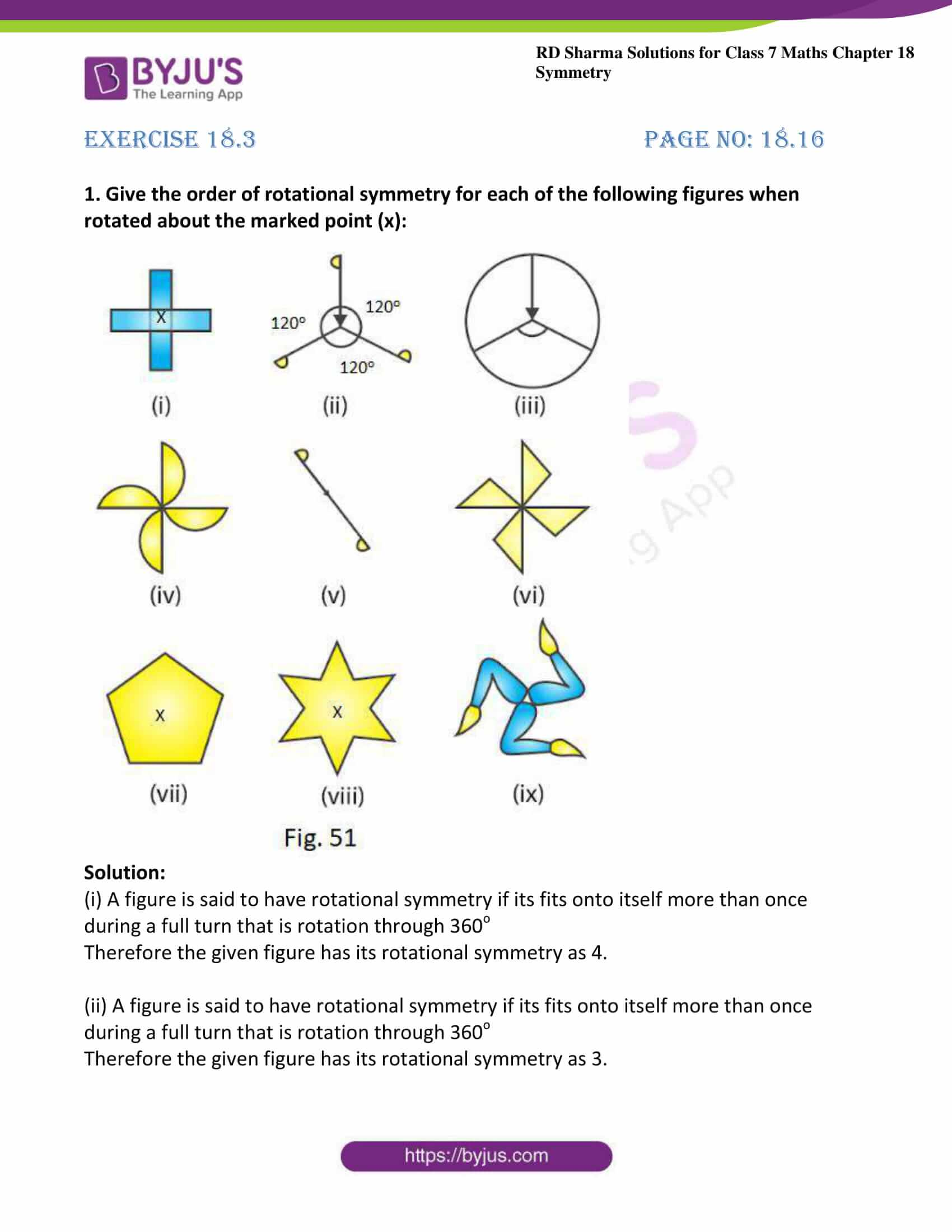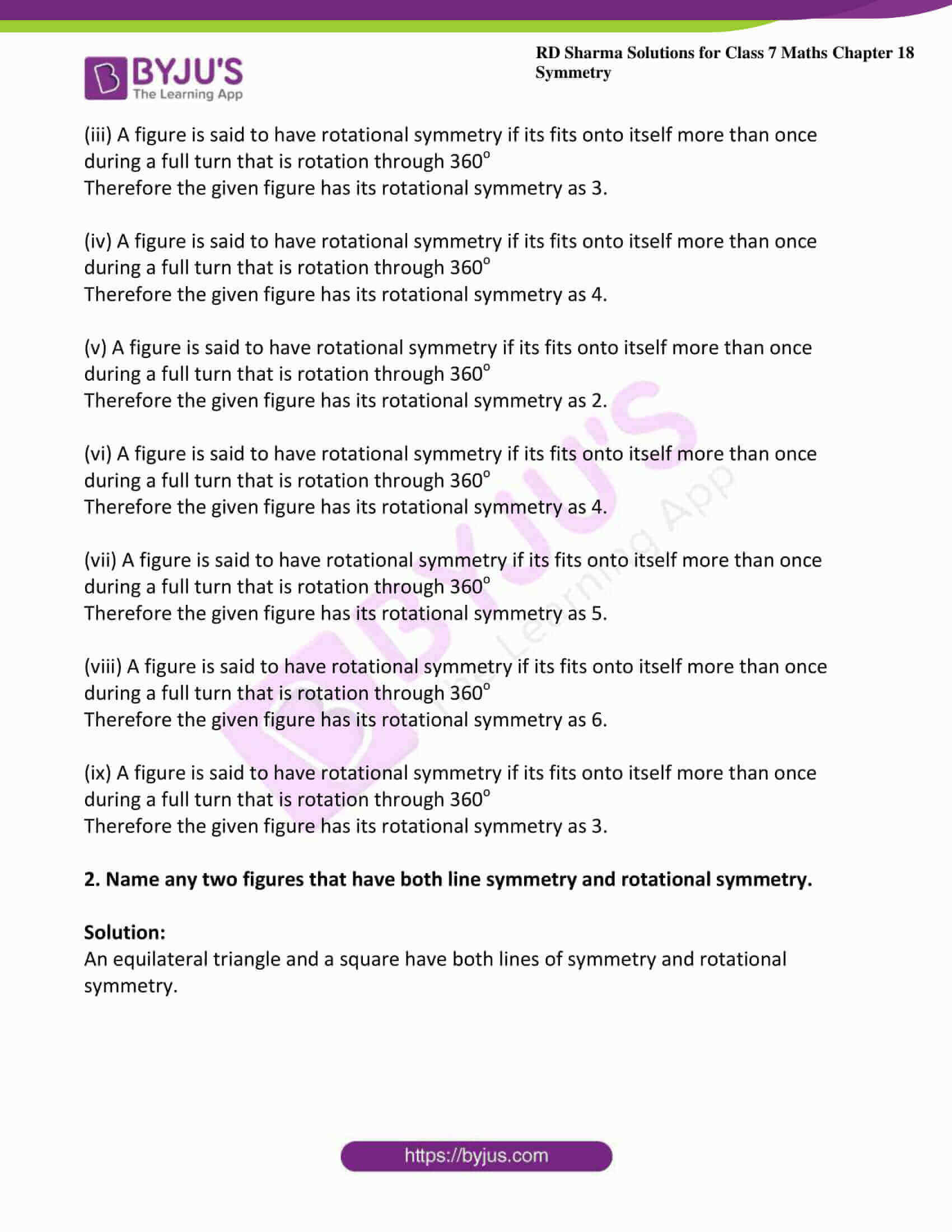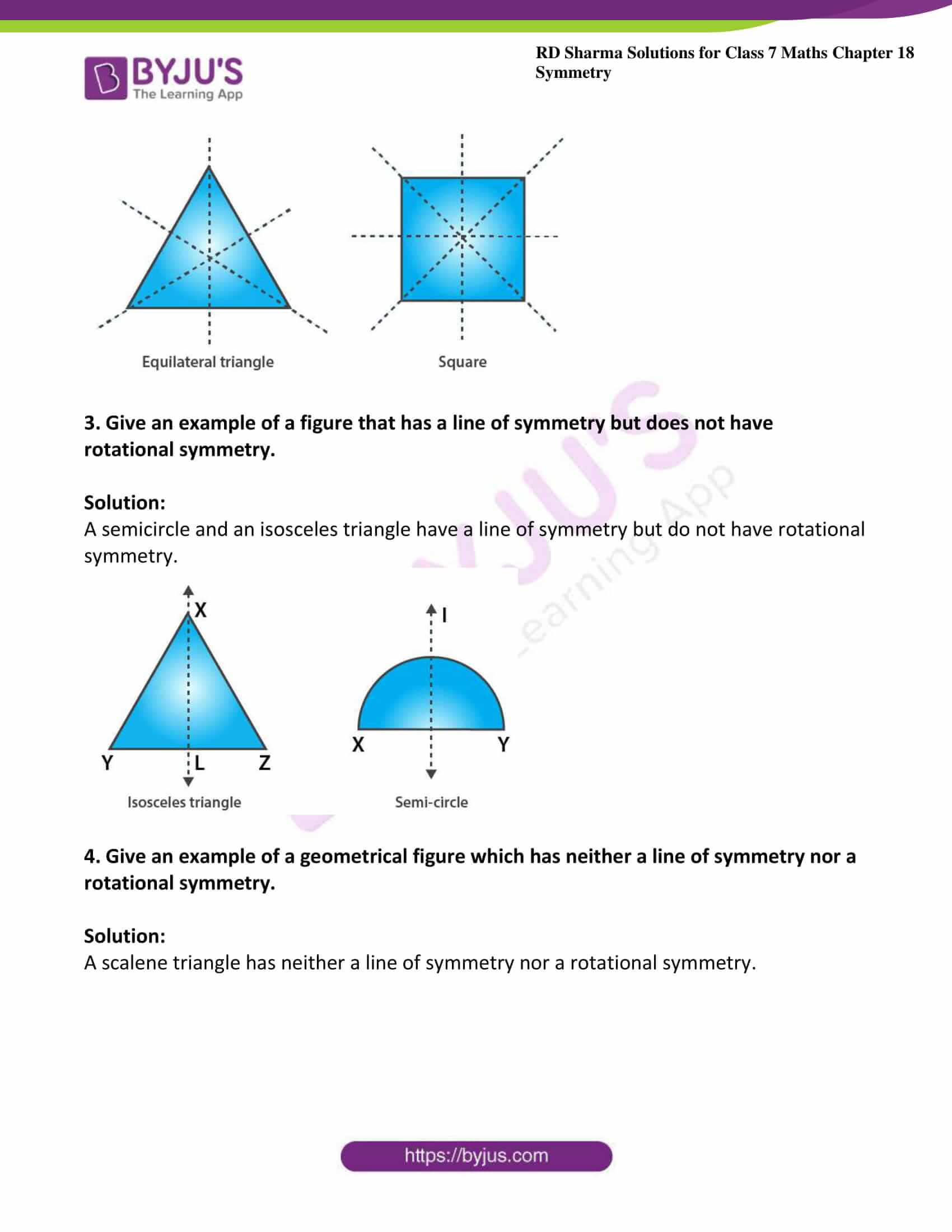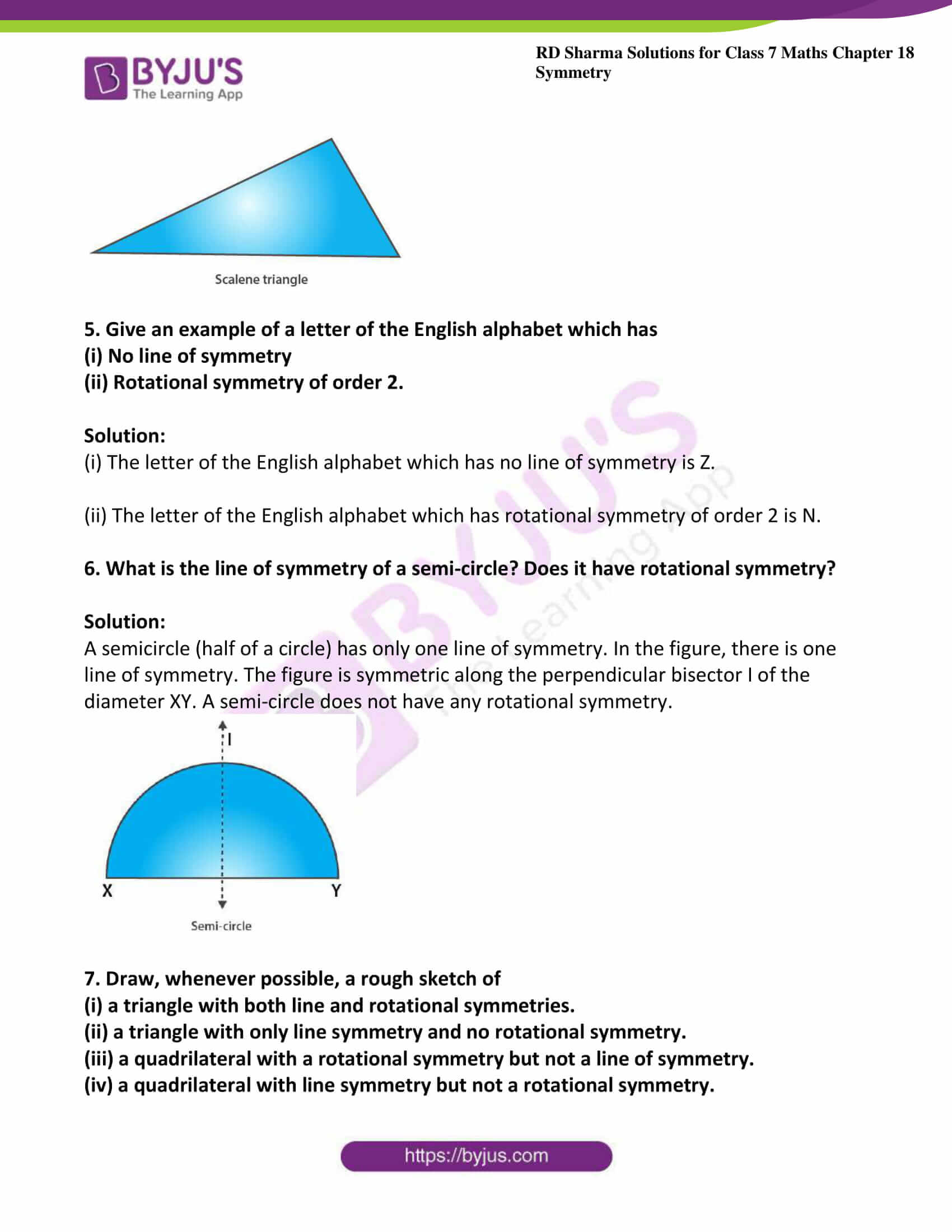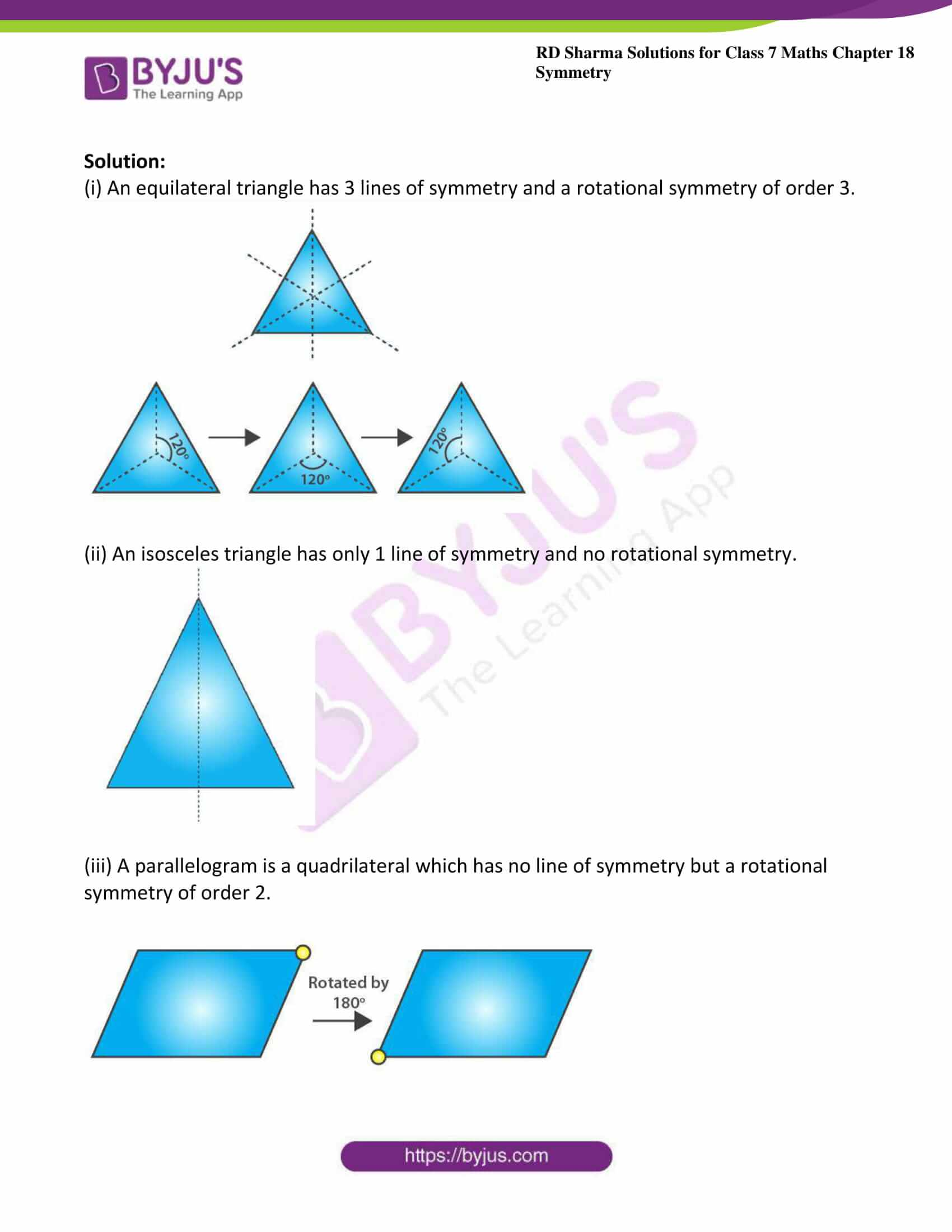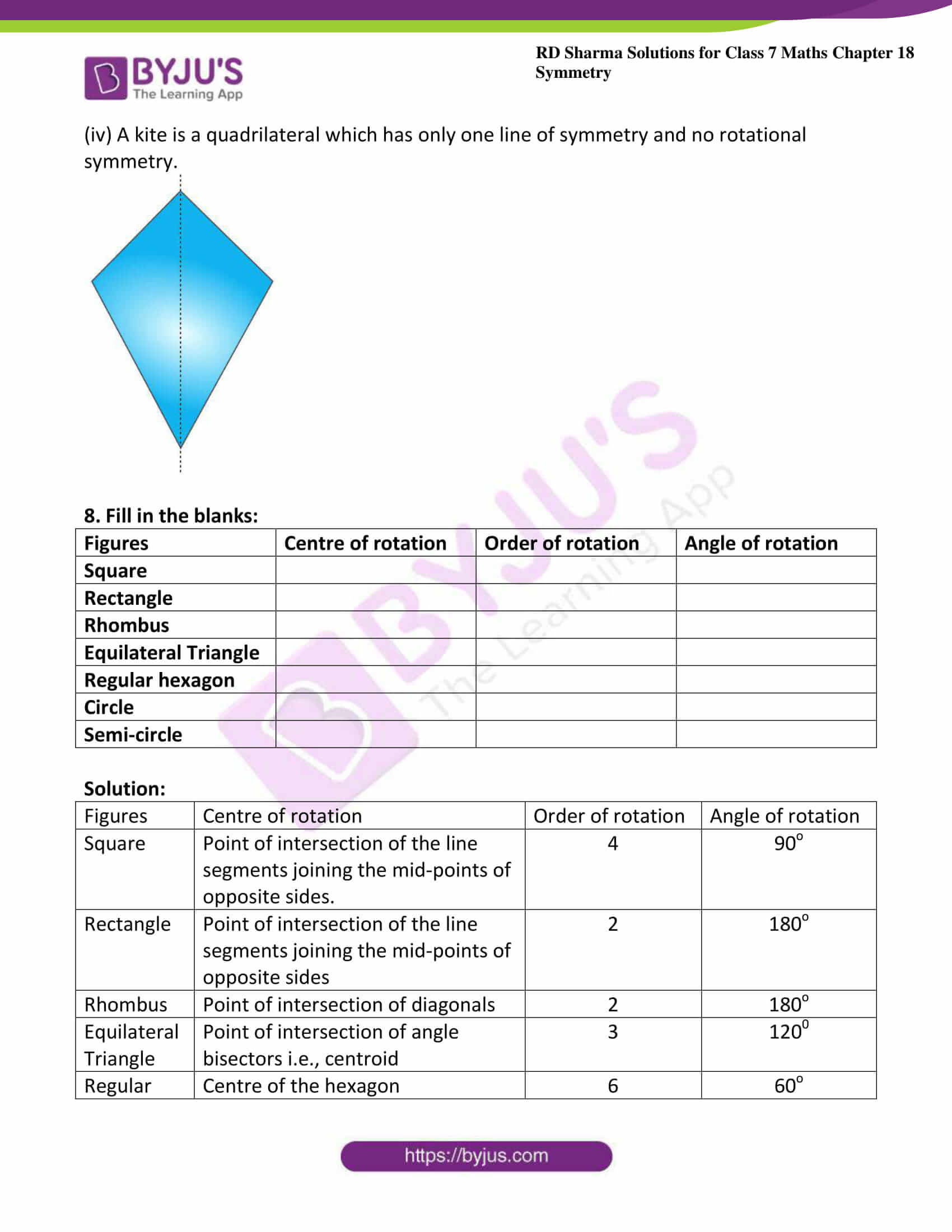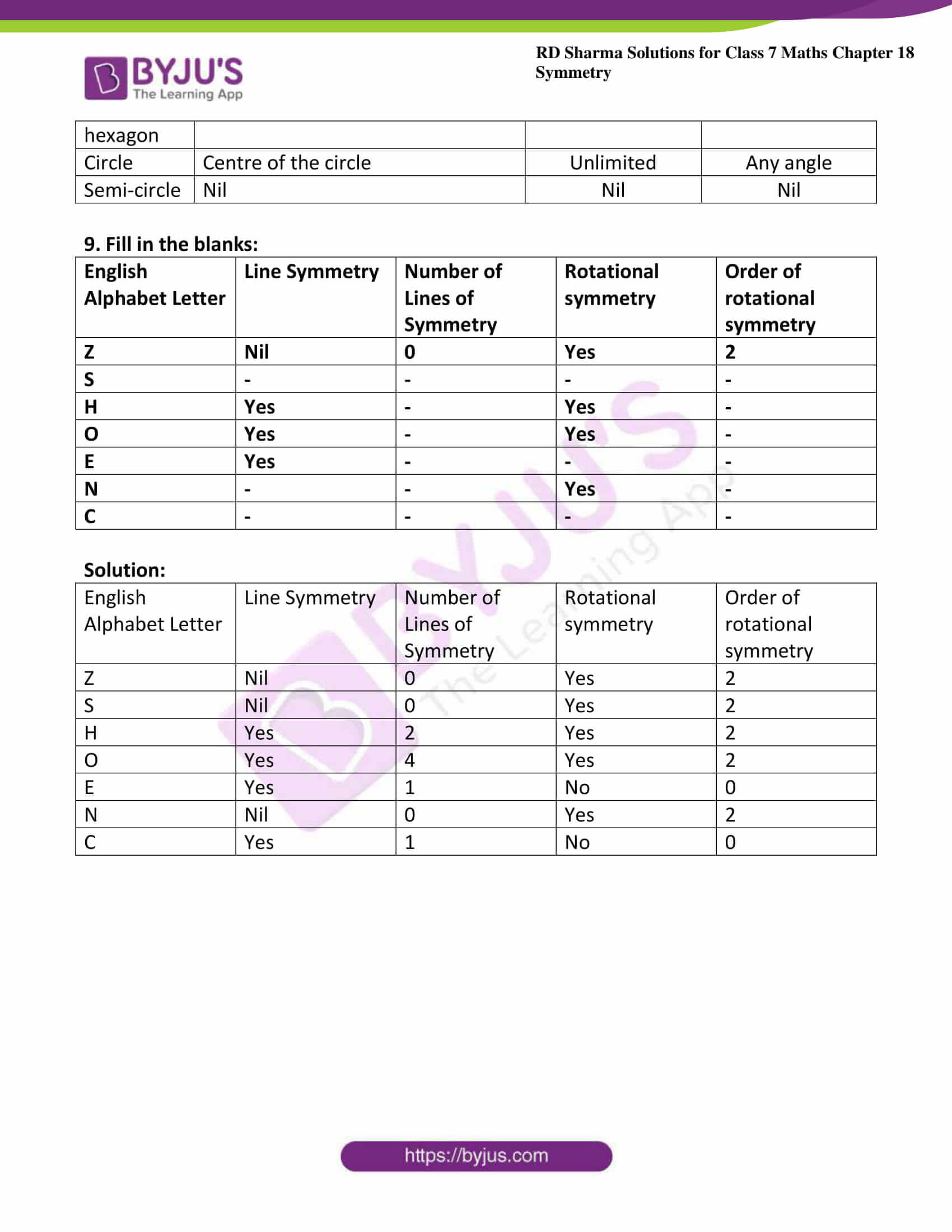### Access answers to Maths RD Sharma Solutions For Class 7 Chapter 18 – Symmetry Exercise 18.3

Exercise 18.3 Page No: 18.16

1. Give the order of rotational symmetry for each of the following figures when rotated about the marked point (x):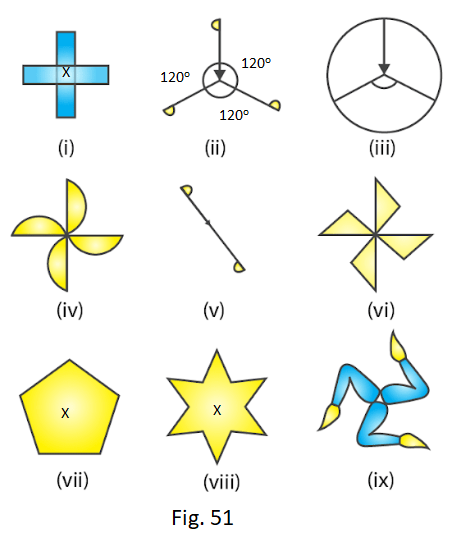Solution:

(i) A figure is said to have rotational symmetry if its fits onto itself more than once during a full turn that is rotation through 360o

Therefore the given figure has its rotational symmetry as 4.

(ii) A figure is said to have rotational symmetry if its fits onto itself more than once during a full turn that is rotation through 360o

Therefore the given figure has its rotational symmetry as 3.

(iii) A figure is said to have rotational symmetry if its fits onto itself more than once during a full turn that is rotation through 360o

Therefore the given figure has its rotational symmetry as 3.

(iv) A figure is said to have rotational symmetry if its fits onto itself more than once during a full turn that is rotation through 360o

Therefore the given figure has its rotational symmetry as 4.

(v) A figure is said to have rotational symmetry if its fits onto itself more than once during a full turn that is rotation through 360o

Therefore the given figure has its rotational symmetry as 2.

(vi) A figure is said to have rotational symmetry if its fits onto itself more than once during a full turn that is rotation through 360o

Therefore the given figure has its rotational symmetry as 4.

(vii) A figure is said to have rotational symmetry if its fits onto itself more than once during a full turn that is rotation through 360o

Therefore the given figure has its rotational symmetry as 5.

(viii) A figure is said to have rotational symmetry if its fits onto itself more than once during a full turn that is rotation through 360o

Therefore the given figure has its rotational symmetry as 6.

(ix) A figure is said to have rotational symmetry if its fits onto itself more than once during a full turn that is rotation through 360o

Therefore the given figure has its rotational symmetry as 3.

2. Name any two figures that have both line symmetry and rotational symmetry.

Solution:

An equilateral triangle and a square have both lines of symmetry and rotational symmetry.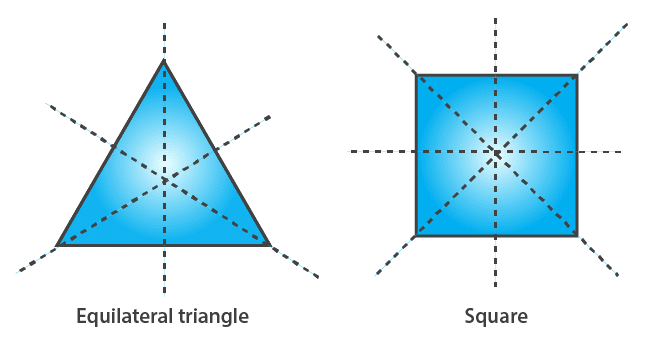3. Give an example of a figure that has a line of symmetry but does not have rotational symmetry.

Solution:

A semicircle and an isosceles triangle have a line of symmetry but do not have rotational symmetry.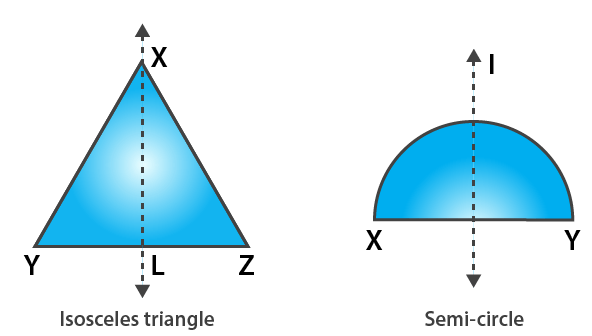4. Give an example of a geometrical figure which has neither a line of symmetry nor a rotational symmetry.

Solution:

A scalene triangle has neither a line of symmetry nor a rotational symmetry.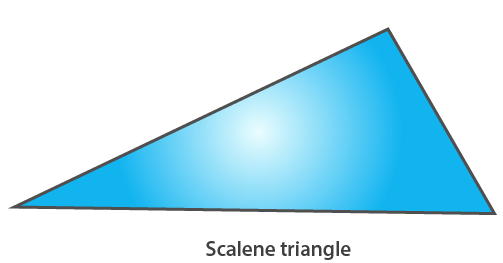5. Give an example of a letter of the English alphabet which has

(i) No line of symmetry

(ii) Rotational symmetry of order 2.

Solution:

(i) The letter of the English alphabet which has no line of symmetry is Z.

(ii) The letter of the English alphabet which has rotational symmetry of order 2 is N.

6. What is the line of symmetry of a semi-circle? Does it have rotational symmetry?

Solution:

A semicircle (half of a circle) has only one line of symmetry. In the figure, there is one line of symmetry. The figure is symmetric along the perpendicular bisector I of the diameter XY. A semi-circle does not have any rotational symmetry.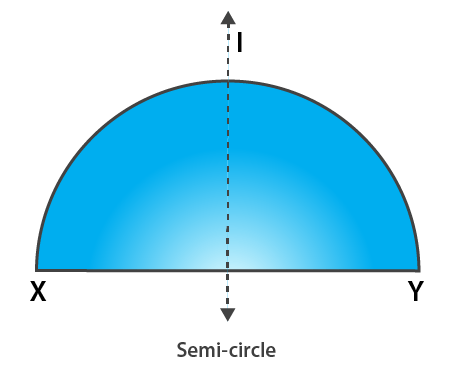7. Draw, whenever possible, a rough sketch of

(i) a triangle with both line and rotational symmetries.

(ii) a triangle with only line symmetry and no rotational symmetry.

(iii) a quadrilateral with a rotational symmetry but not a line of symmetry.

(iv) a quadrilateral with line symmetry but not a rotational symmetry.

Solution:

(i) An equilateral triangle has 3 lines of symmetry and a rotational symmetry of order 3.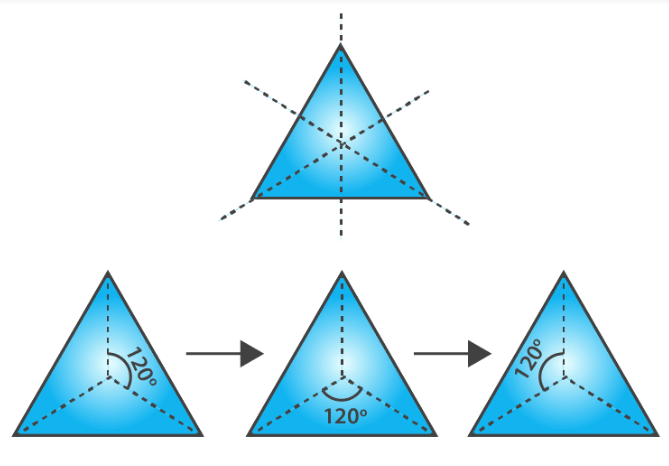(ii) An isosceles triangle has only 1 line of symmetry and no rotational symmetry.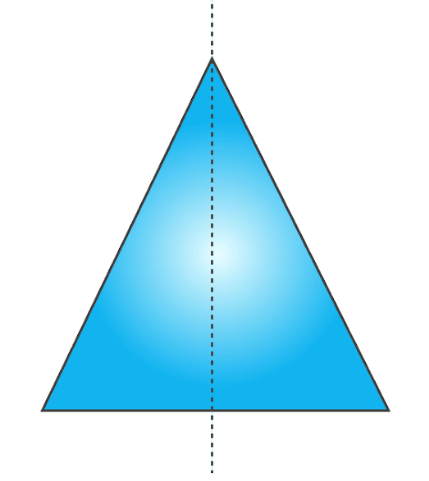(iii) A parallelogram is a quadrilateral which has no line of symmetry but a rotational symmetry of order 2.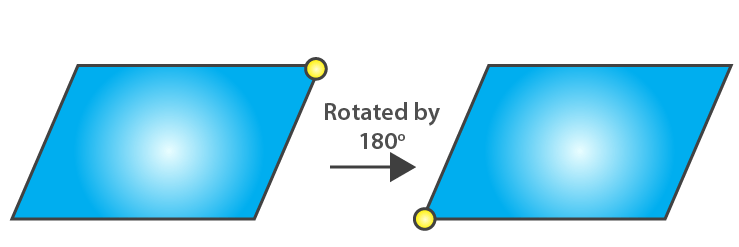(iv) A kite is a quadrilateral which has only one line of symmetry and no rotational symmetry.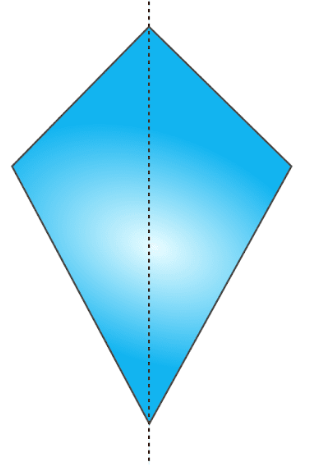8. Fill in the blanks:

 Figures Centre of rotation Order of rotation Angle of rotation Square Rectangle Rhombus Equilateral Triangle Regular hexagon Circle Semi-circle

Solution:

 Figures Centre of rotation Order of rotation Angle of rotation Square Point of intersection of the line segments joining the mid-points of opposite sides. 4 90o Rectangle Point of intersection of the line segments joining the mid-points of opposite sides 2 180o Rhombus Point of intersection of diagonals 2 180o Equilateral Triangle Point of intersection of angle bisectors i.e., centroid 3 1200 Regular hexagon Centre of the hexagon 6 60o Circle Centre of the circle Unlimited Any angle Semi-circle Nil Nil Nil

9. Fill in the blanks:

 English Alphabet Letter Line Symmetry Number of Lines of Symmetry Rotational symmetry Order of rotational symmetry Z Nil 0 Yes 2 S – – – – H Yes – Yes – O Yes – Yes – E Yes – – – N – – Yes – C – – – –

Solution:

 English Alphabet Letter Line Symmetry Number of Lines of Symmetry Rotational symmetry Order of rotational symmetry Z Nil 0 Yes 2 S Nil 0 Yes 2 H Yes 2 Yes 2 O Yes 4 Yes 2 E Yes 1 No 0 N Nil 0 Yes 2 C Yes 1 No 0